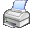﻿ bDataColumn:CalculateWidth()

# bDataColumn:CalculateWidth()Purpose

Calculates the optimal width of the data column.

Class

bDataColumn

Type

Method

Syntax

<oDataColumn>:CalculateWidth() Æ iWdth

Return Value

 iWidth The calculated width of the column.
 Data Type: DWord

Description

bDataColumn:CalculateWidth() calculates the optimal width of the column, dependent on the field that is linked with the column, its column caption and its column footer.

The calculated width is set automatically in the bDataColumn object and can be queried at a later time by the access bBrowserColumn:Width.

So that changes at the column width can be processed correct by the bBrowser, the browser must be recalculated by calling the method bBrowser:Recalculate(). This is only necessary if the column is inside the visible area of the browser.